# Three sets of 2022 Python level 10 exam questions and answer analysis

‘### Preface

I haven’t logged in on Douban for a long time. I saw the “Breaking Bad Grade 10 Scholar Unified Exam” posted by [Zhu Xiaoluzhu] the day before yesterday. I didn’t answer the questions and found it to be quite interesting. Then, I thought of the topic “Python Grade 10 Exam”. ，Find some Python-related, common but likely to be wrongly answered questions and write them into test papers. As a result, I found that I had enough three test questions, so I wrote three consecutive articles on the WeChat public account:

These topics are mainly inspired by wtfpython and the Tweet of Mike Driscoll, the author of “Mouse Vs Python”, of course, I also added some personal goods of my own.

The third question will be a little more difficult. If you want to try it, you can go in and write the answer through the link of the official account article. After I’m done, look at the answers and analysis of this question I wrote later.

In addition, if you didn’t answer the question I marked [Send points], I suggest you find a time to focus on learning Python again.

### Topic 1

Look at the 10 questions of National Volume A first.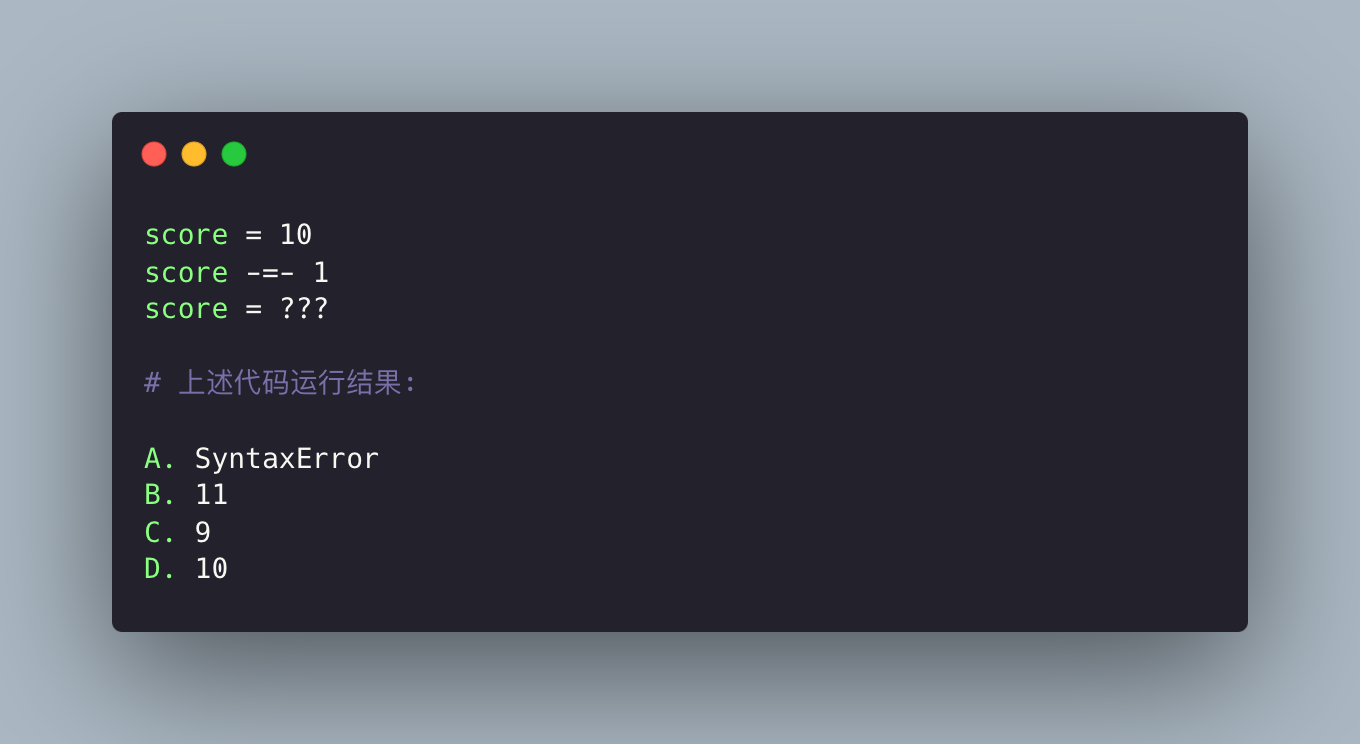This thread comes from a Tweet by Raymond Hettinger.

The answer is B. Because `- 1` (with a space in the middle) is actually `-1` , which means that `score -= (-1)` can be expressed.

### Topic 2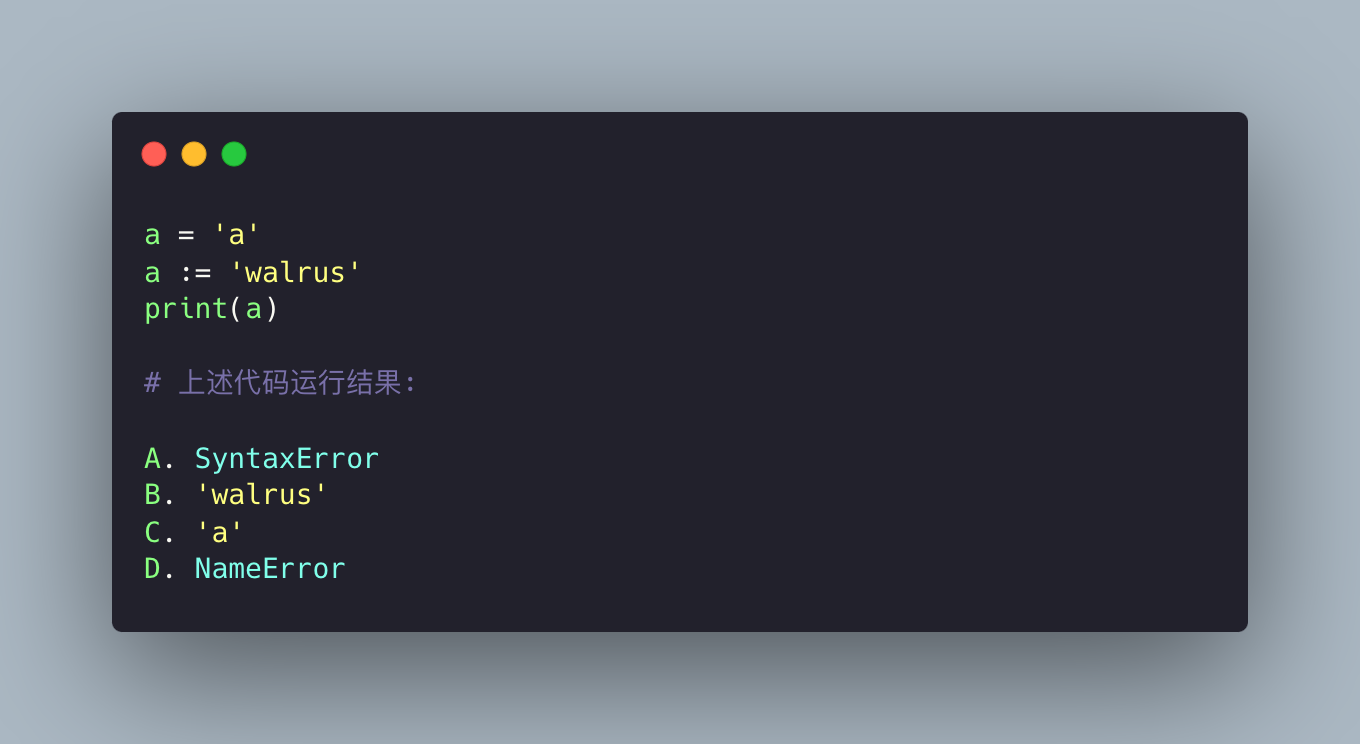For the sub-question, the answer is A, that is, a SyntaxError error is thrown, because the walrus operator needs to use parentheses and cannot be used directly, because it needs to be distinguished from ordinary assignment.

### topic 3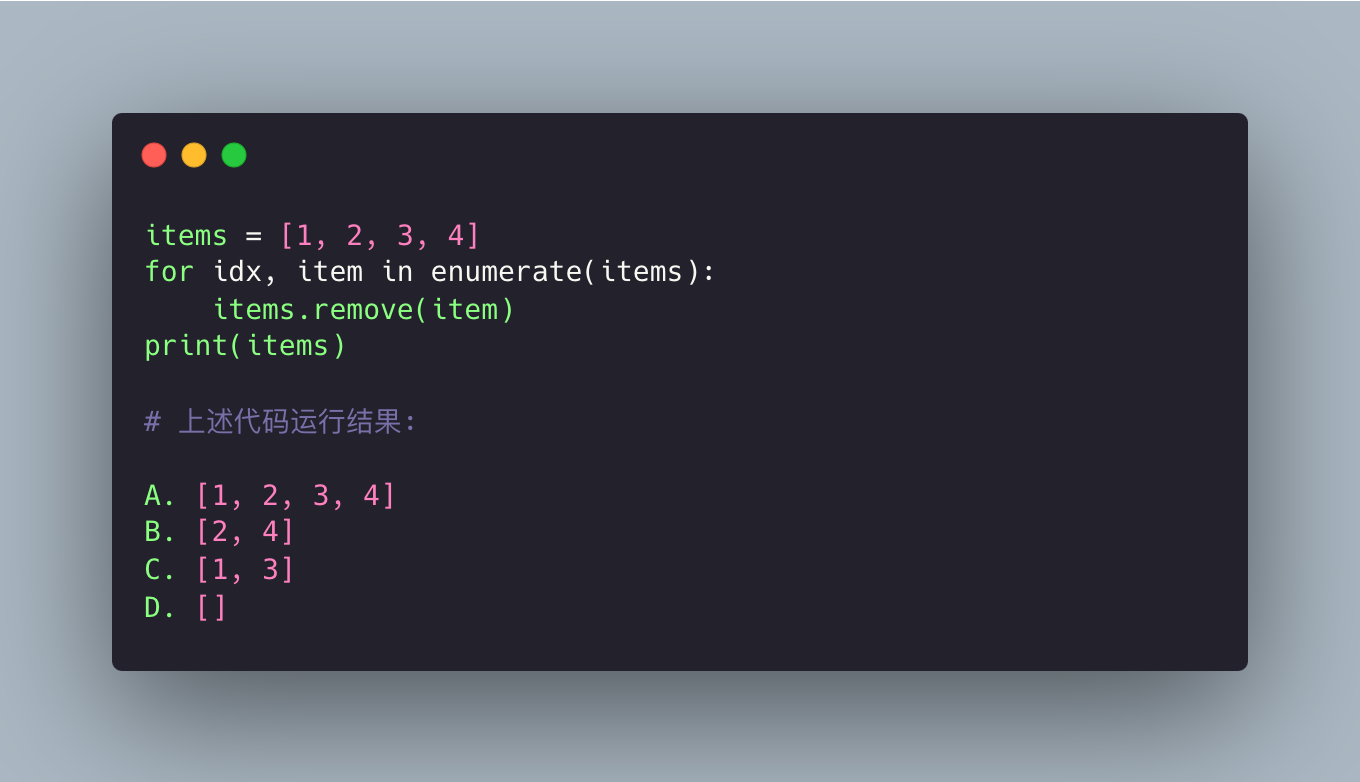This topic is mainly to test the understanding of iteration. In the loop, the first element 1 (index 0) is iterated first and then remove deletes this element, leaving three elements 2, 3, 4, but note that the index of 2 is 0 and the index of 3 is 1. The next iteration should be index 1, which is to iterate and delete 3, skip 2, and then skip 4. Anything skipped is left, so the result is `[2, 4]` .

### Topic 4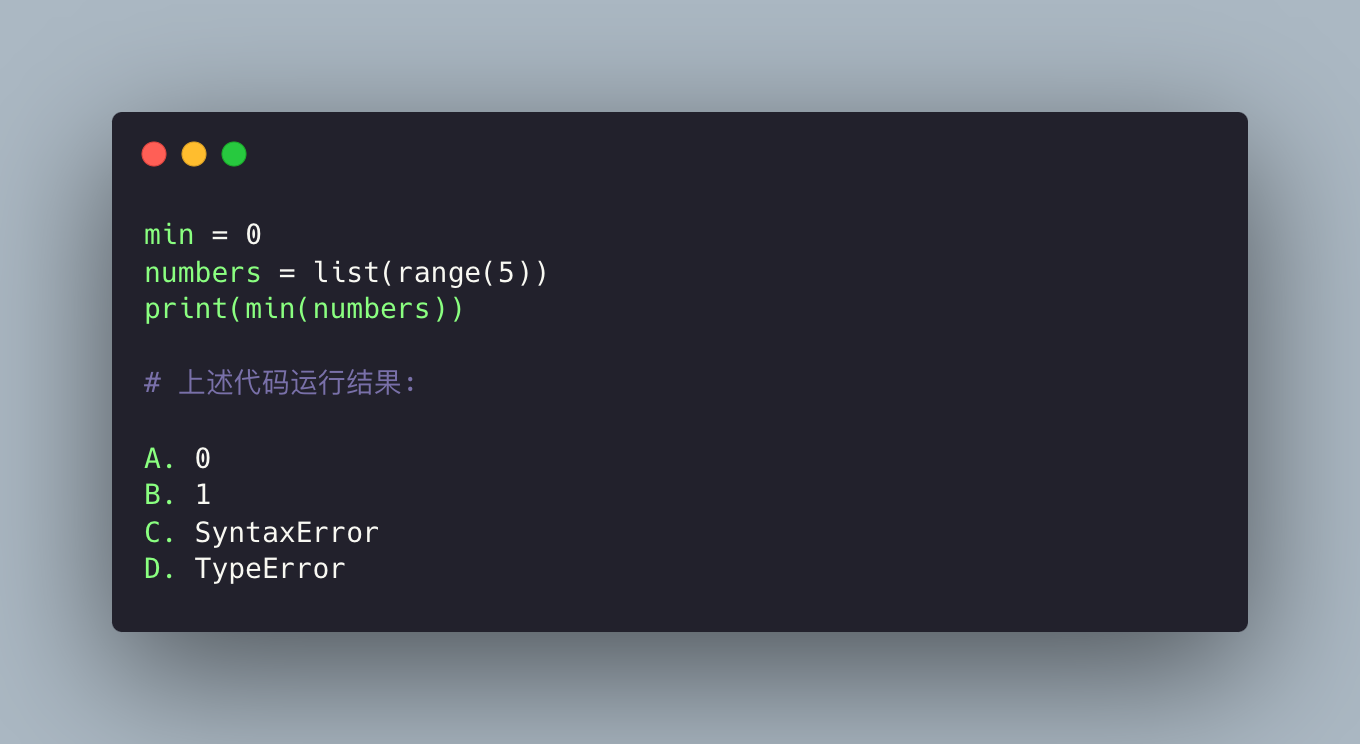For the sub-question, the answer is D, because `min` is a built-in function. If you replace it with other objects, it will not work properly, and a TypeError will be thrown.

### topic 5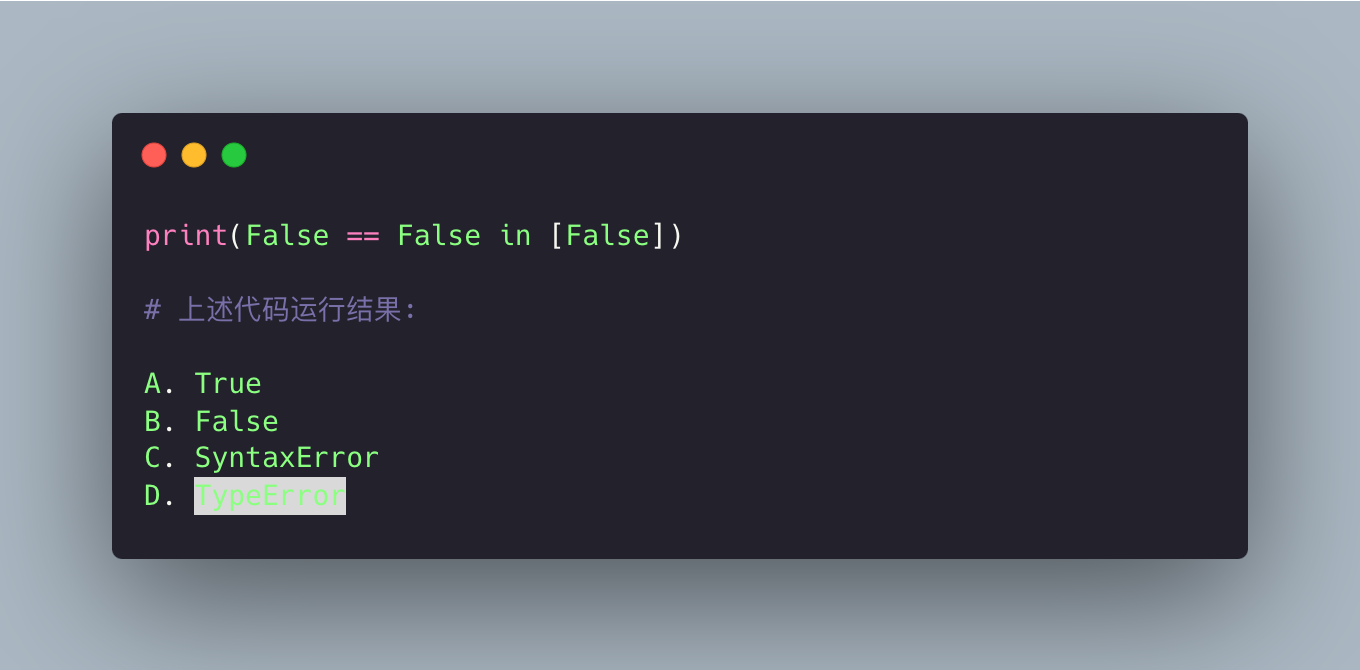The answer is A, which is particularly counterintuitive, right? But it should be noted that the comparison method is to compare the two adjacent ones in order. The official website says :

if a, b, c, …, y, z are expressions and op1, op2, …, opN are comparison operators, then a op1 b op2 c … y opN z is equivalent to a op1 b and b op2 c and … y opN z, except that each expression is evaluated at most once.

So `False == False in [False]` means `(False == False) and (False in [False])` , so the result is True.

### topic 6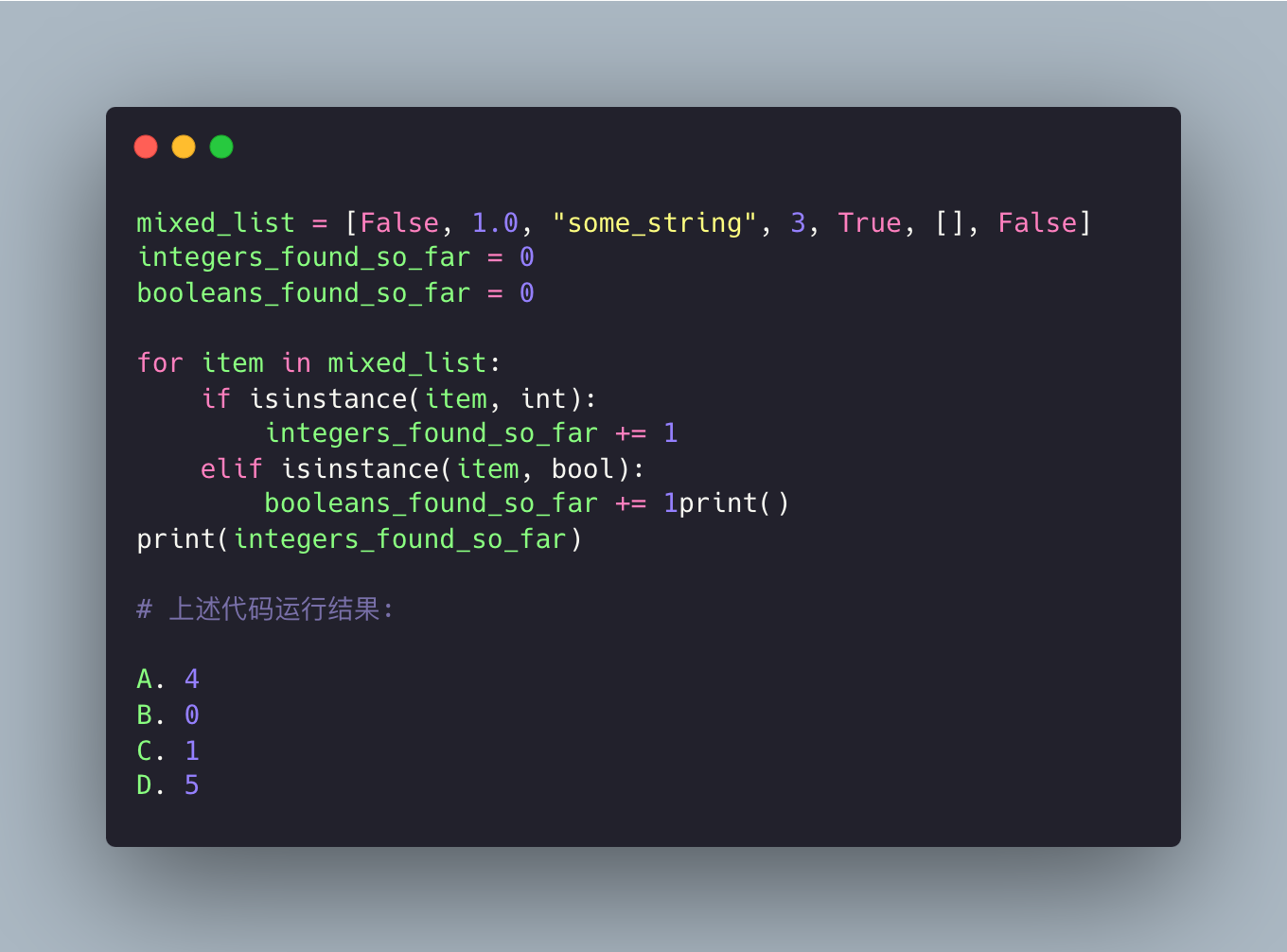For the sub-question, the answer is A, because the bool value is also a number (True is 1, False is 0):

 ` In : isinstance ( True , int ) Out : True In : 'haha' * True Out : 'haha' In : 'haha' * False Out : '' `

### topic 7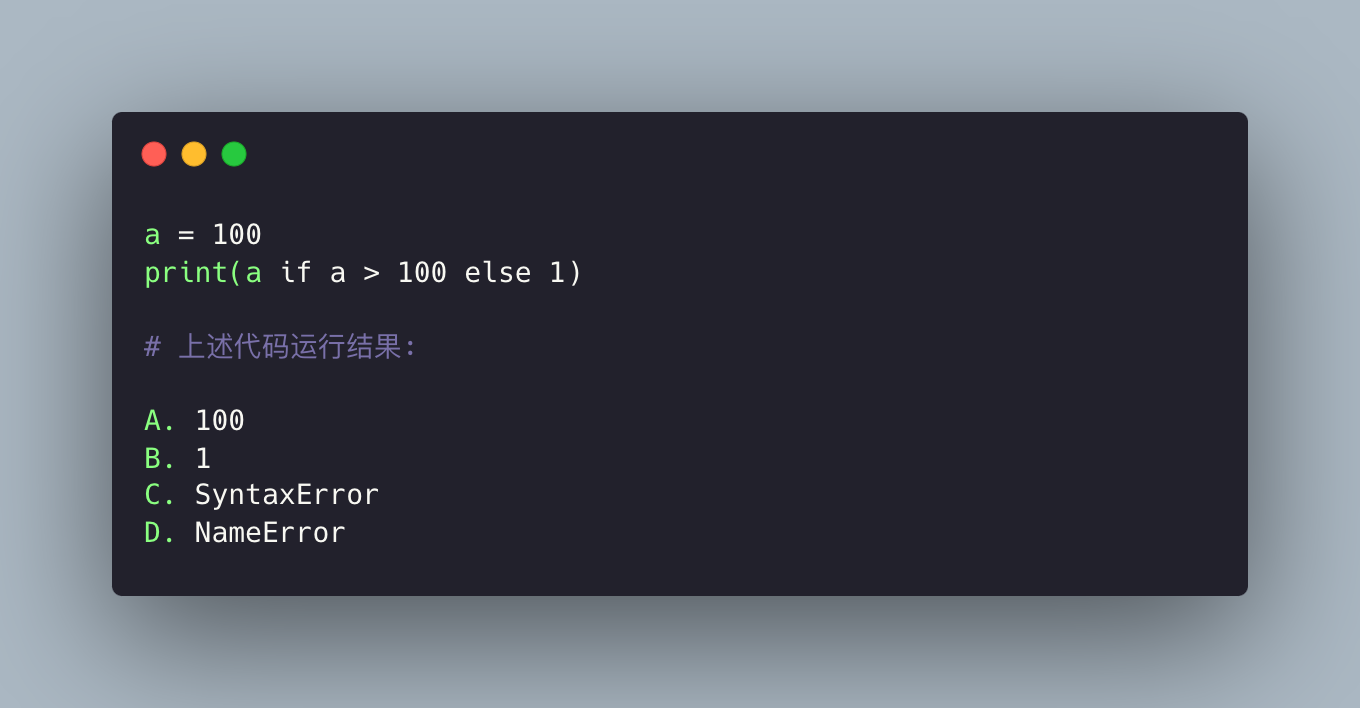The answer is B. In this question, I just want everyone to know that the judgment can be written directly in the print, instead of this:

 ` In : a = 100 In : result = a if a > 100 else 1 In : print ( result ) 1 `

### topic 8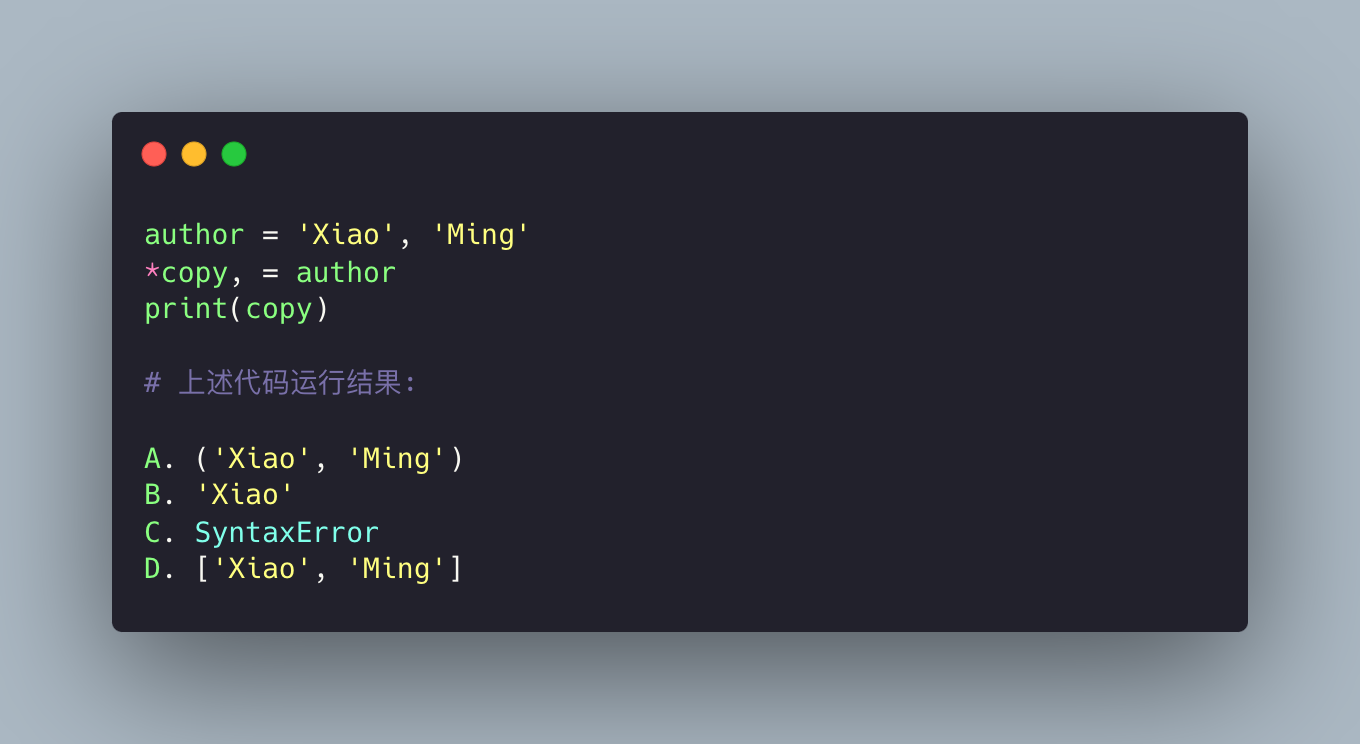For the sub-question, the answer is D, and the knowledge point is Unpacking.

### topic 9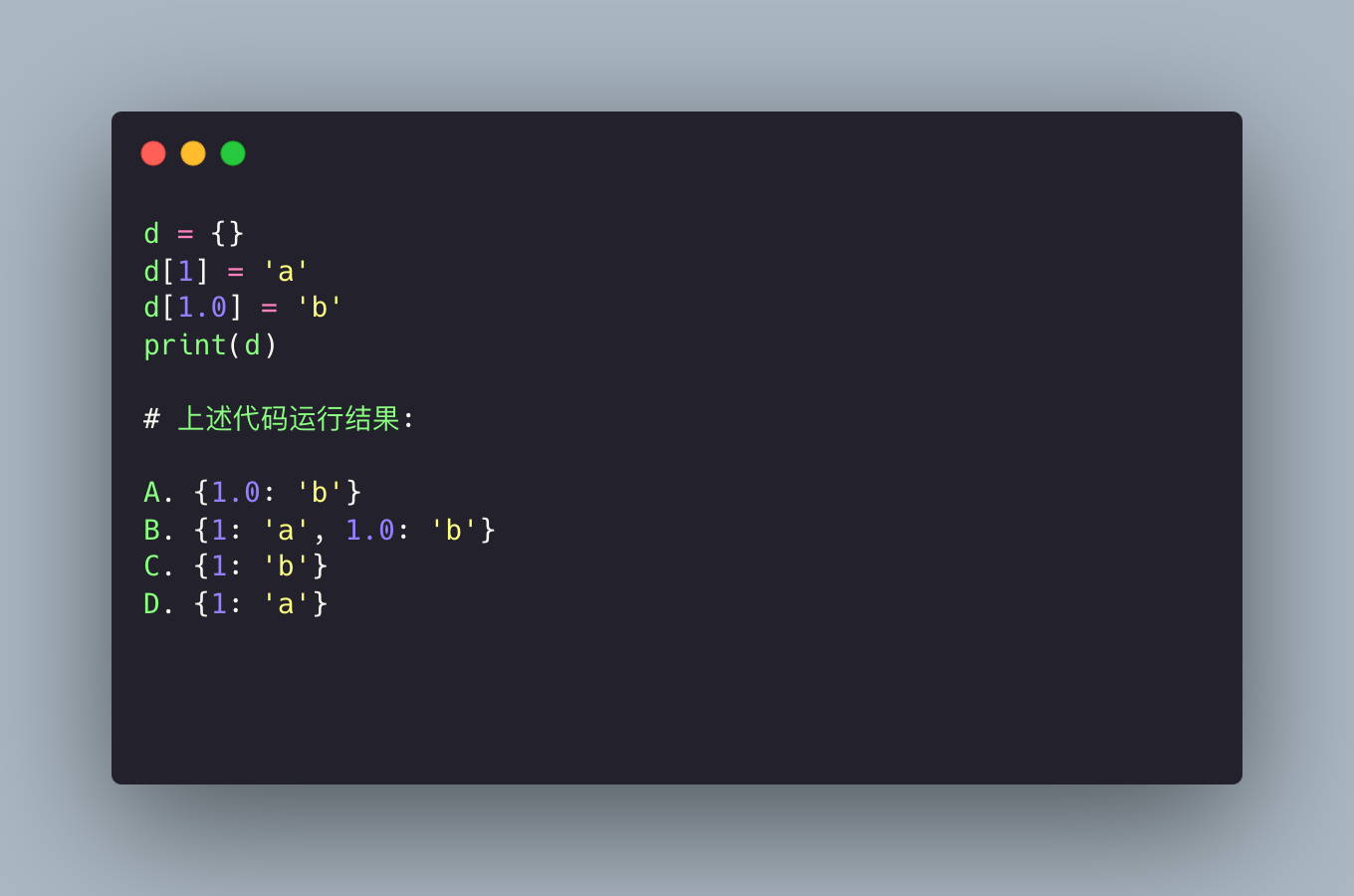The answer is C. In Python’s dictionary, it doesn’t care about the type of the keys, as long as their values ​​are the same, they are the same key-value pair, and subsequent assignments replace the previous values:

 ` In : 1 == 1.0 Out : True `

### topic 10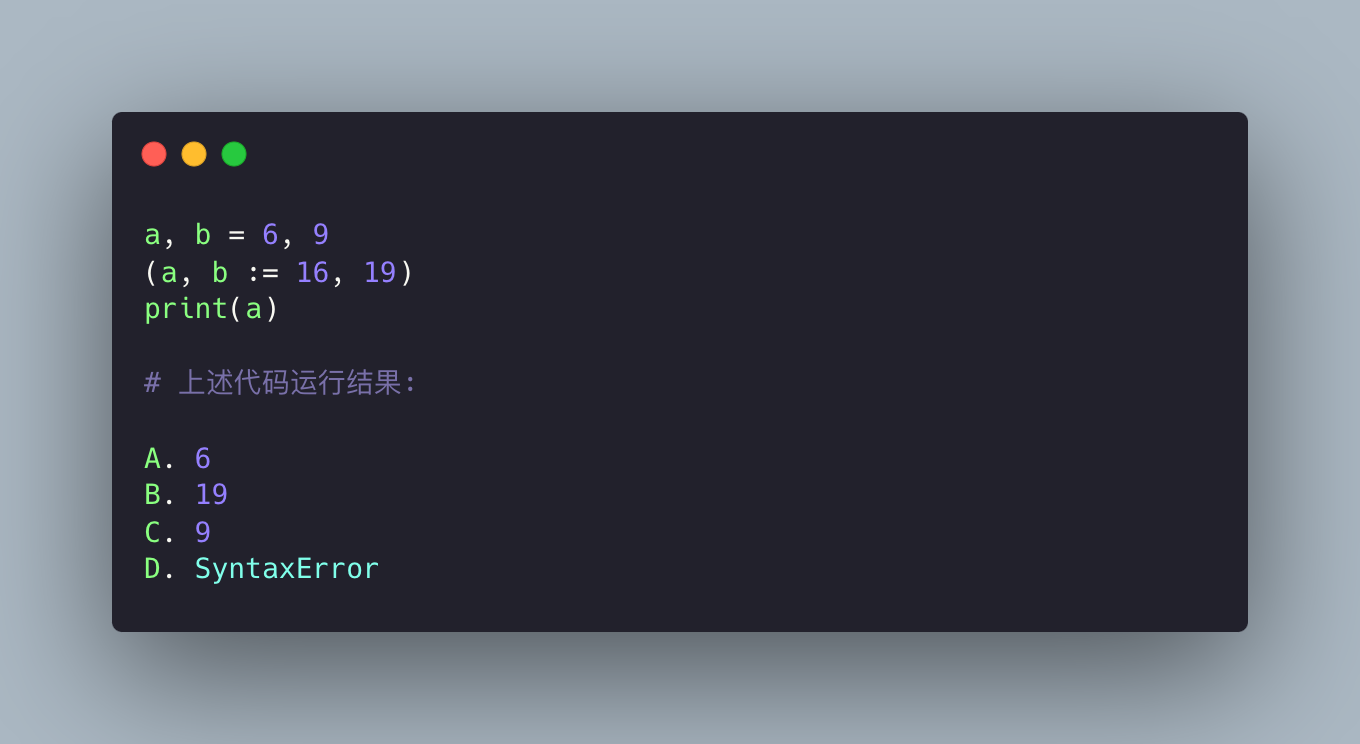The answer is A, the source can’t be found, I also wrote an article about this confusing code using the walrus operator

### topic 11

Look again at the 10 questions in National Volume B.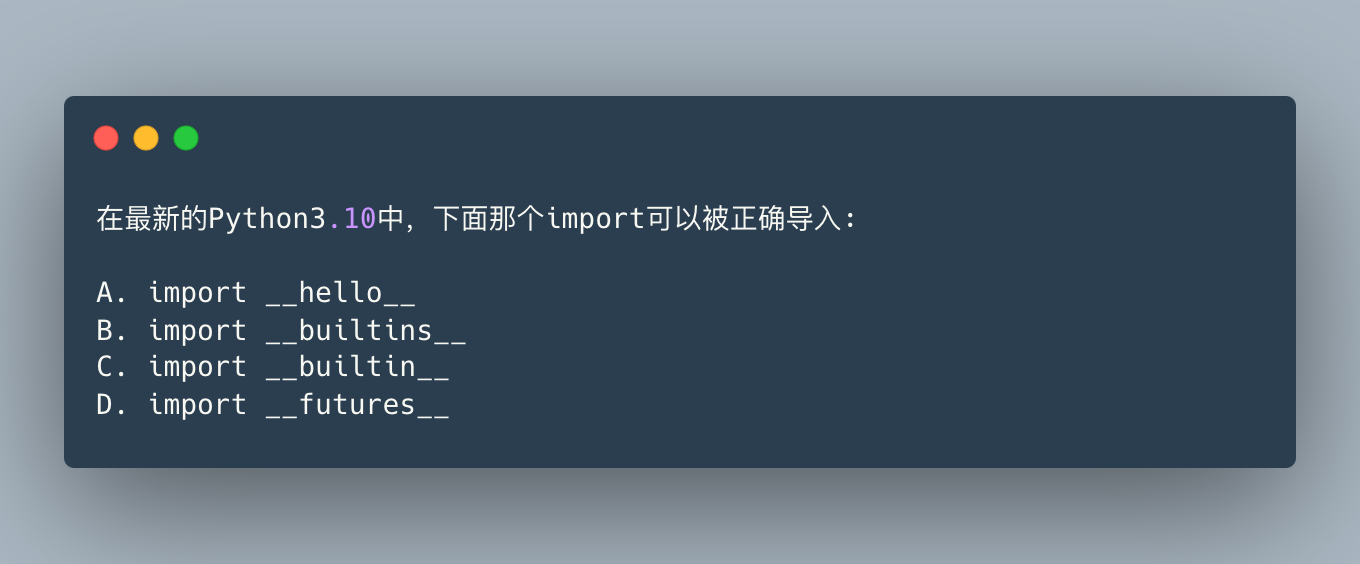This is more difficult to understand, the answer is A. This is the module that Python freeze automatically creates, in addition to `__phello__` :

 ` In : import __hello__ Hello world ! In : import __phello__ Hello world ! In : import __phello__ # Only the first import will be executed, and then it will be [cached]. `

Among other options, `__builtin__` is a module from the Python 2 era, and there is a builtins module that can be used by Python2/3, but there is no `__builtins__` . In addition, there are `__future__` and `futures` but no `__futures__` , which are used for confusion.

### topic 12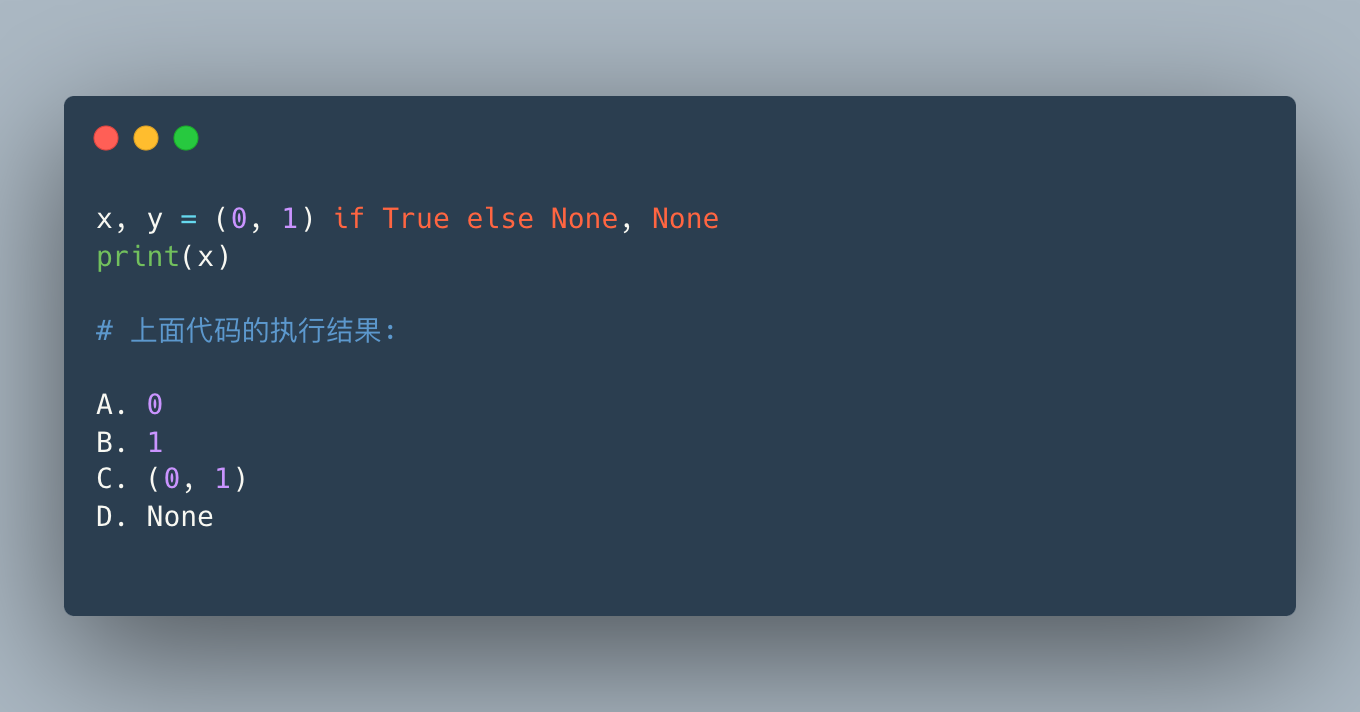The answer is C. This is very similar to question 10. You can think of it as an assignment statement, the one before the comma is assigned to x, and the one after the comma is assigned to y. If you add parentheses, it means another way:

 ` In : x , y = ( 0 , 1 ) if True else ( None , None ) In : x Out : 0 In : y Out : 1 `

This means that different tuples are assigned according to the judgment conditions.

### topic 13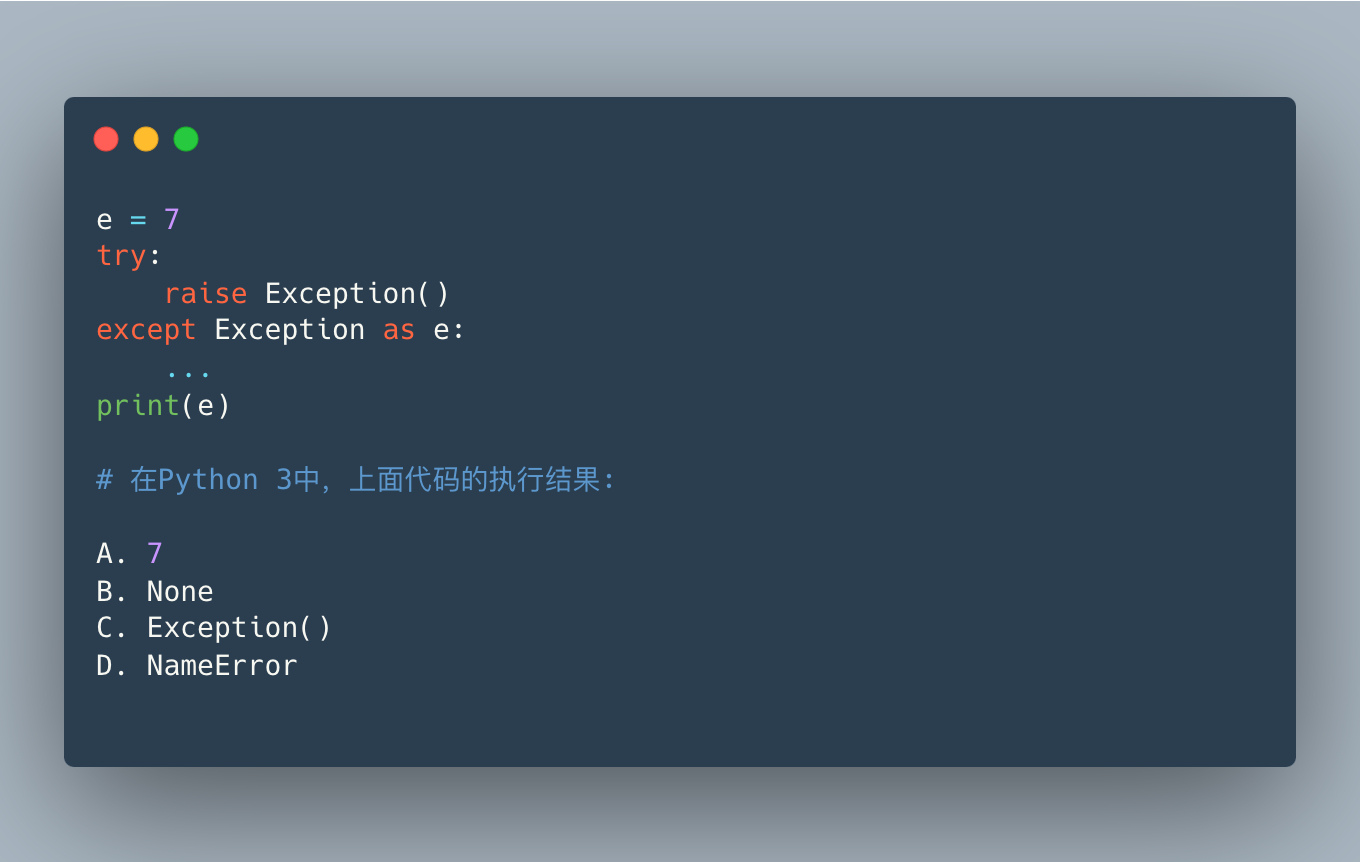The answer is D. In Python2, the result of e is `Exception()` . Note that this does not match the description in the wtfpython project.

Why throw NameError directly in Python3? because:

 ` except E as N : foo `

In the except scope it is actually equivalent to:

 ` except E as N : try : foo finally : del N `

That is to say, the alias N of as will be deleted eventually when leaving the scope, so that e does not exist, so it is a NameError.

### topic 14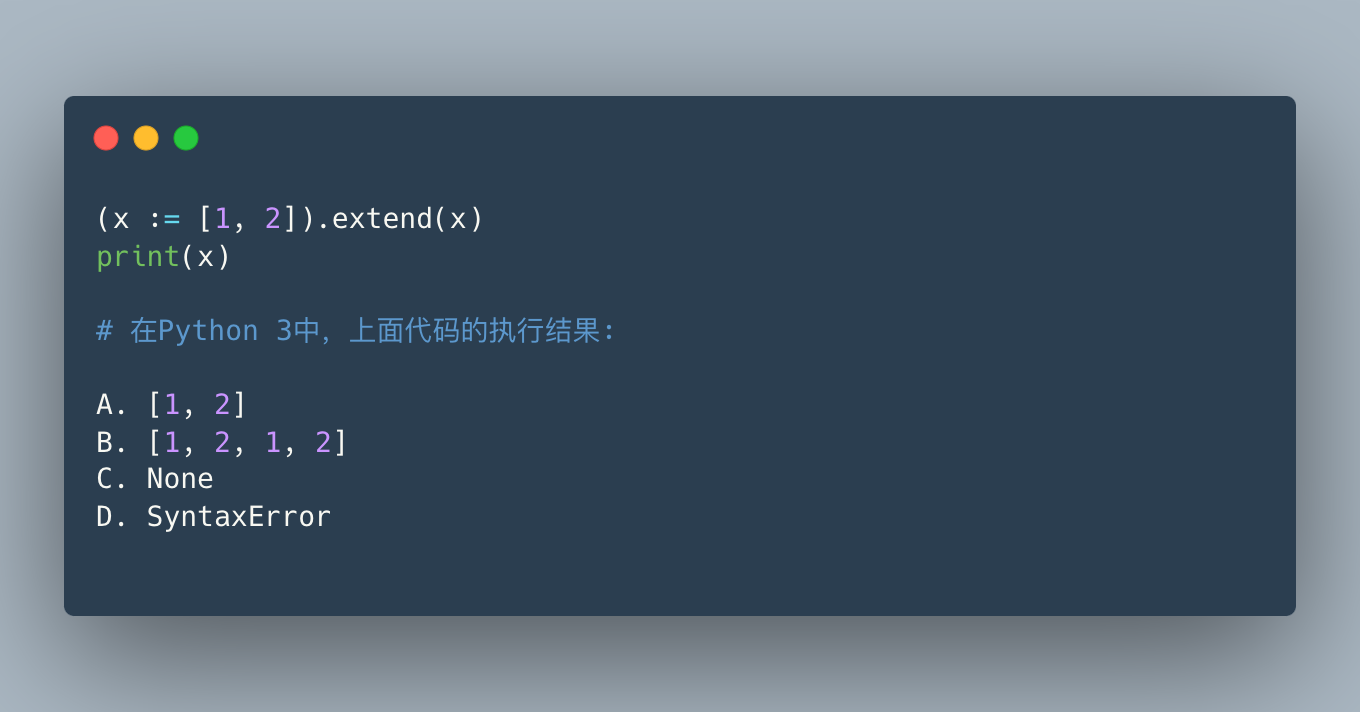Give points, the answer is B. This is a common application scenario of the walrus operator. First, assign x to `[1, 2]` in `(x := [1, 2])` , and then execute `x.extend(x)` on this x.

### topic 15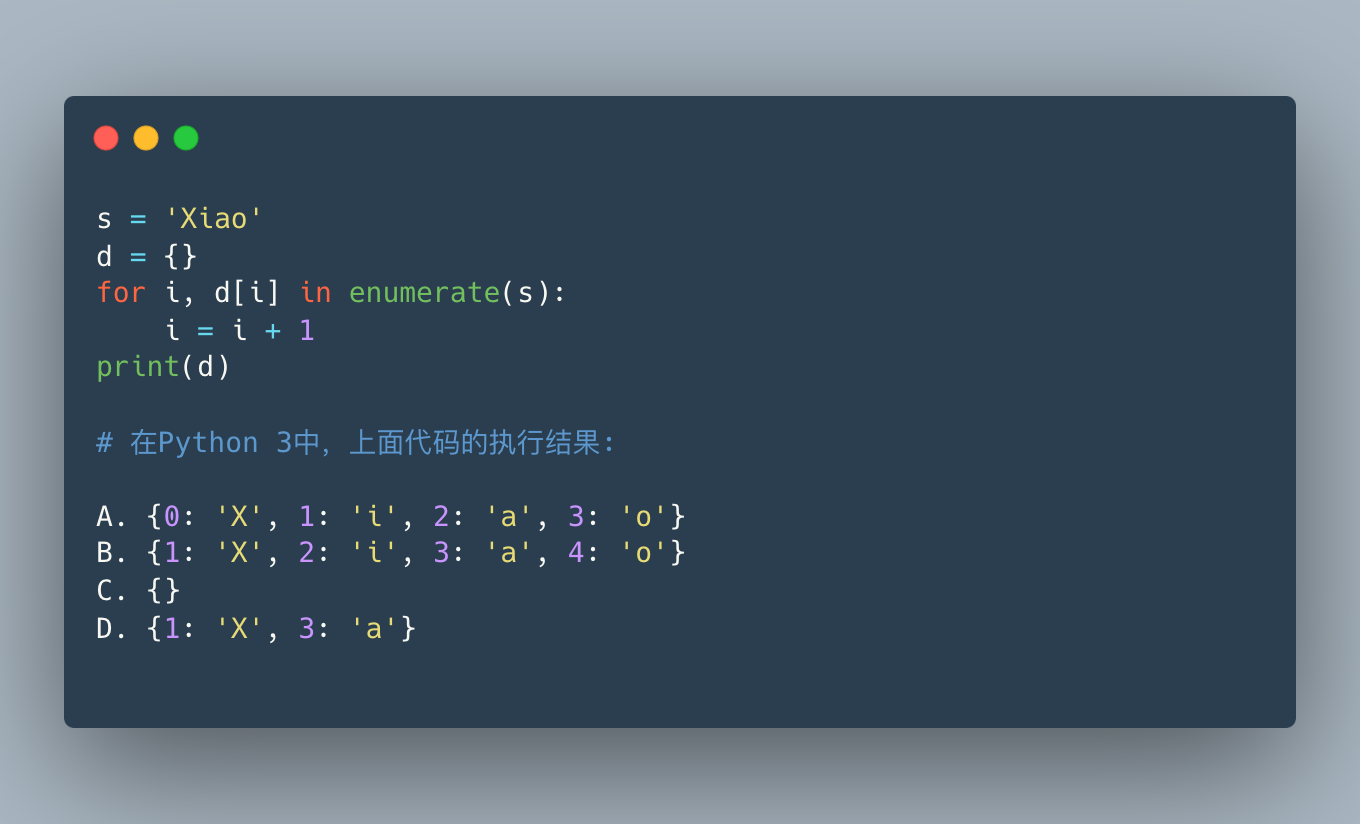The answer is A. This topic is to show that there are many other things you can do while looping, such as assigning values ​​to iterative objects by the way. I posted a similar Douban broadcast a long time ago: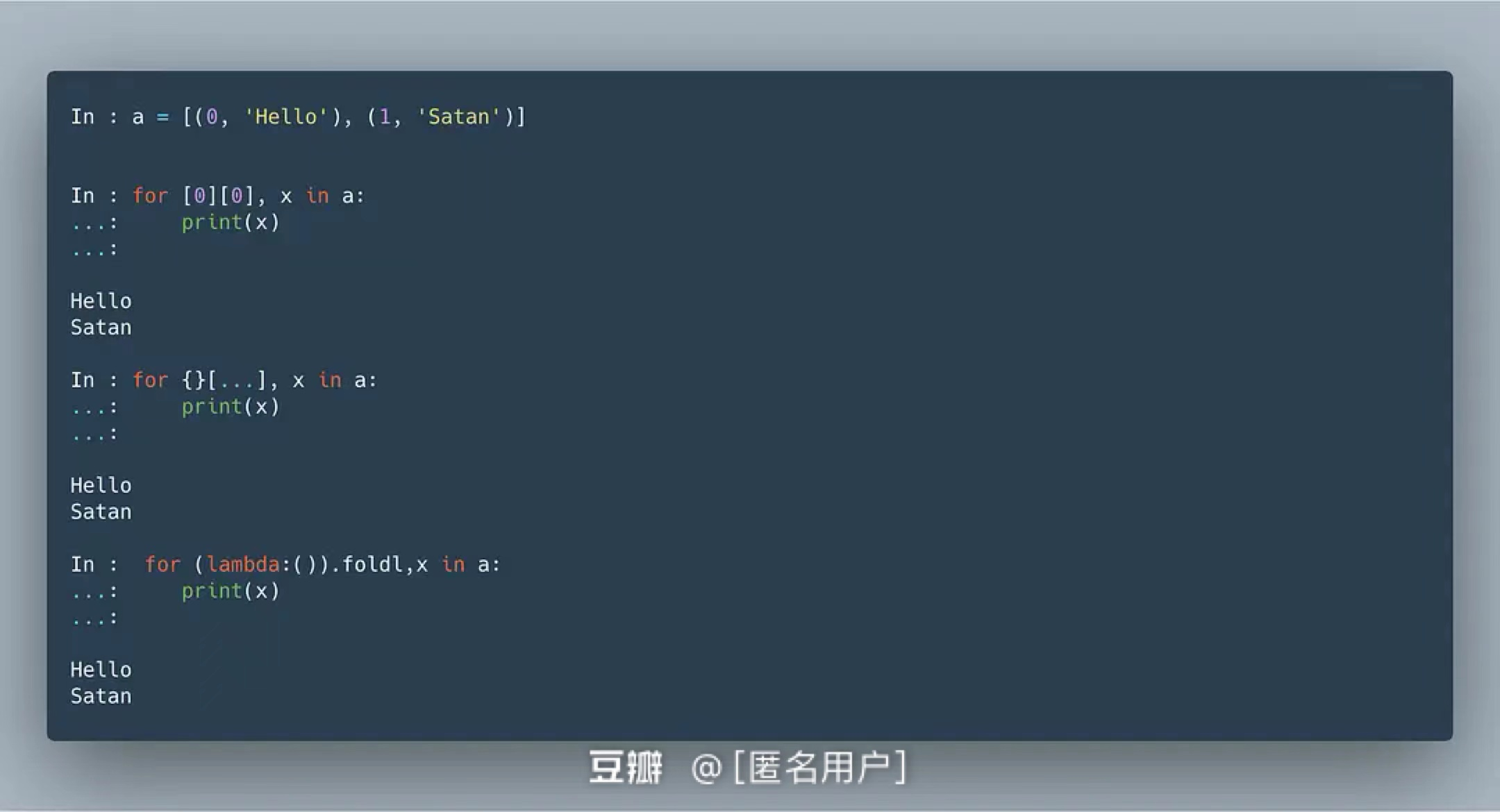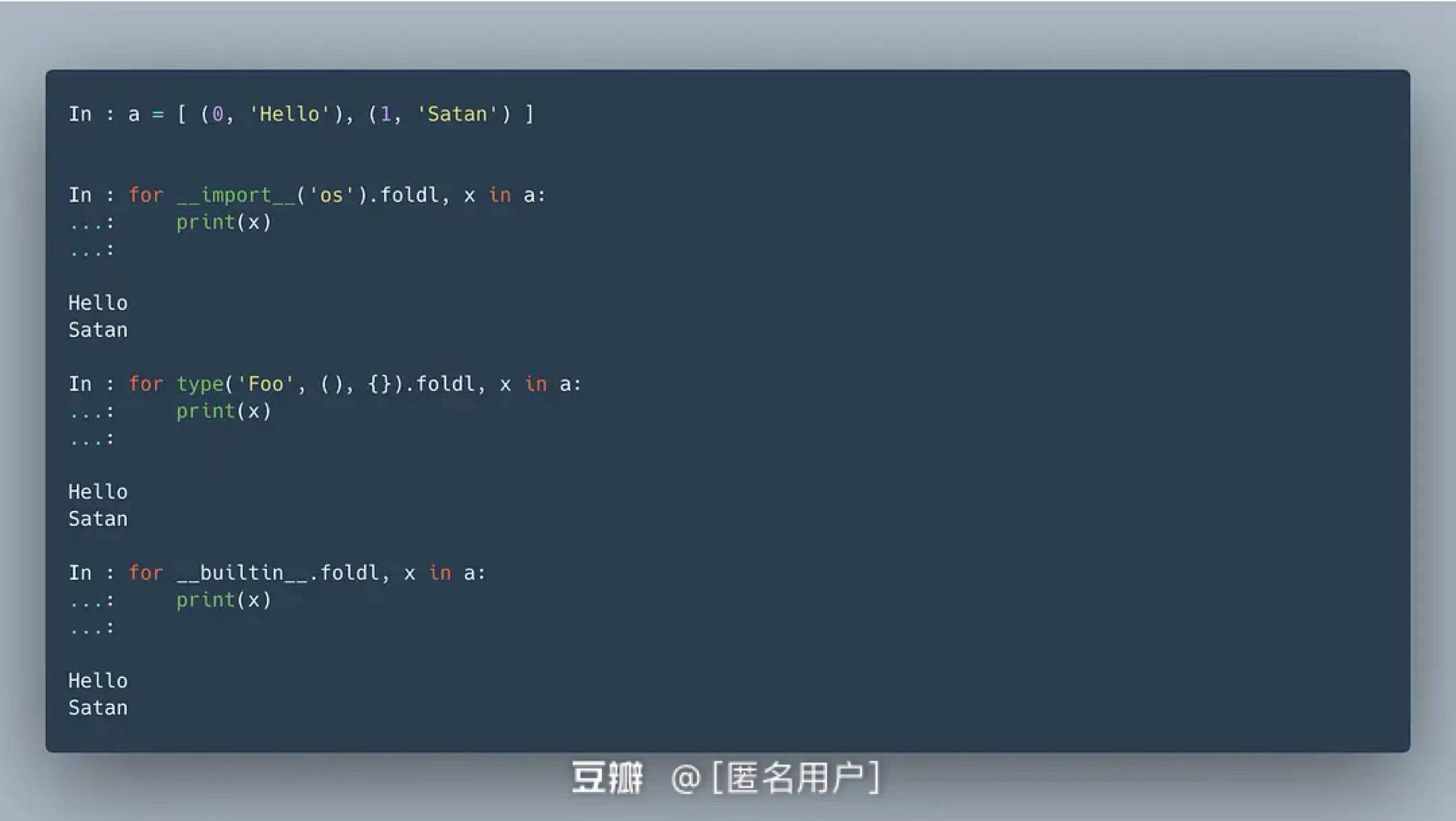### Topic 16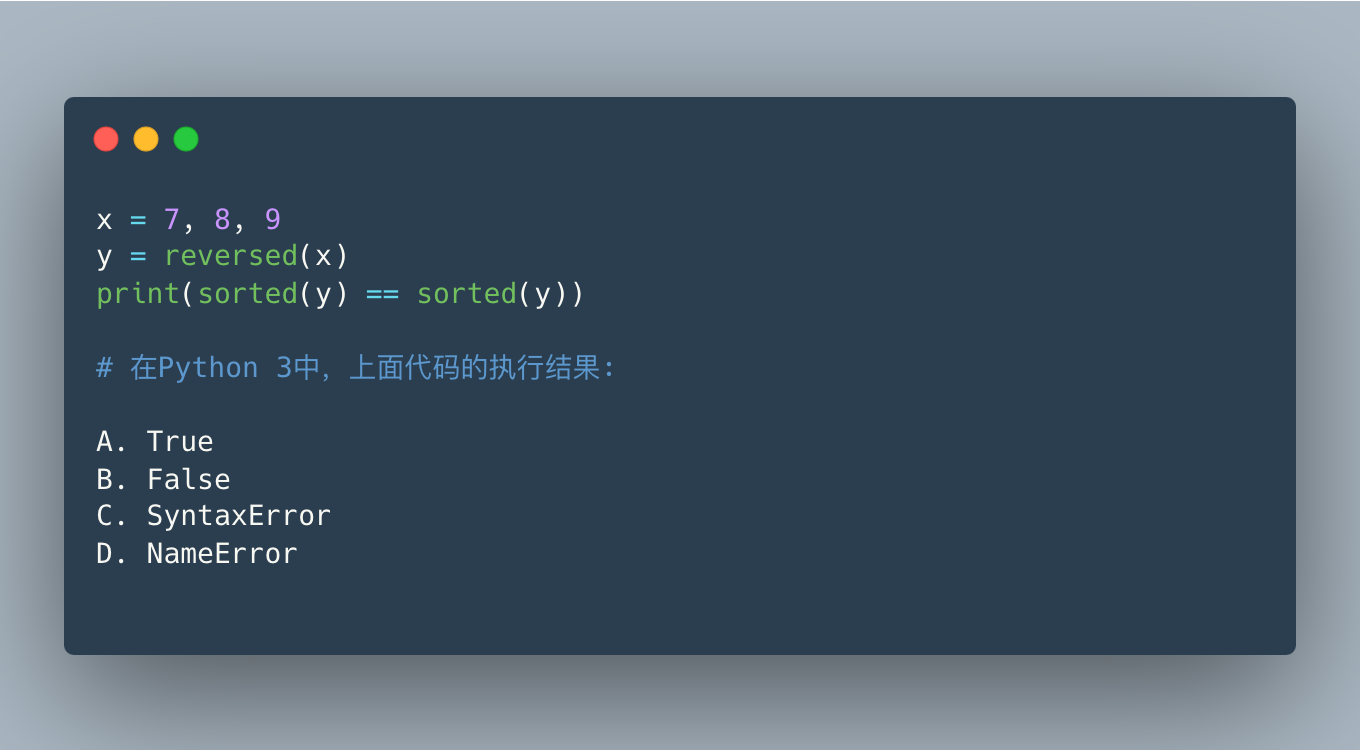The answer is B. I got it wrong the first time. The problem is that when you iterate over an iterable object twice, the iterative object is already empty at the beginning of the next iteration:

 ` In : x = 7 , 8 , 9 In : y = reversed ( x ) In : sorted ( y ), sorted ( y ) Out : ([ 7 , 8 , 9 ], []) `

### Topic 17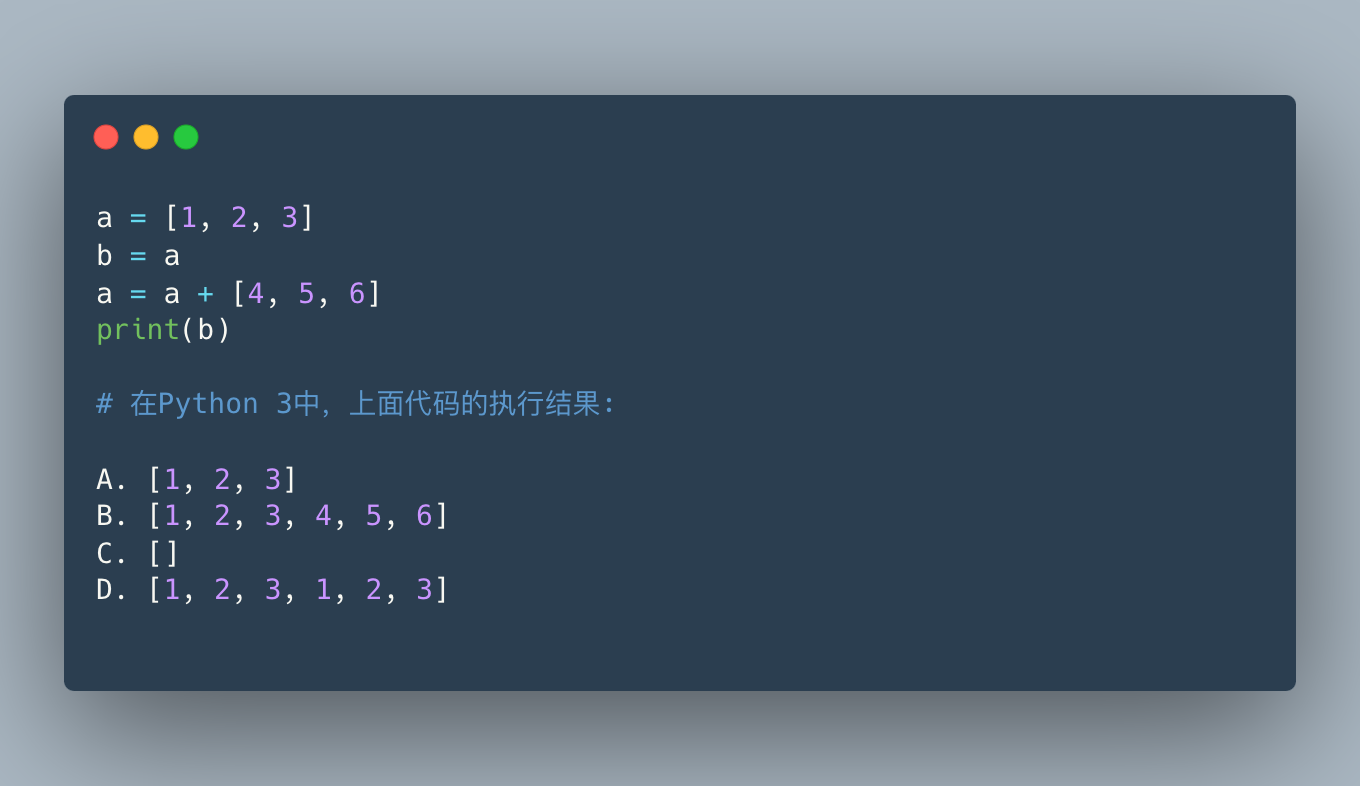Give points, the answer is A. `a += [4, 5, 6]` will generate a new list a, but b refers to the old a, so it won’t be affected.

### Topic 18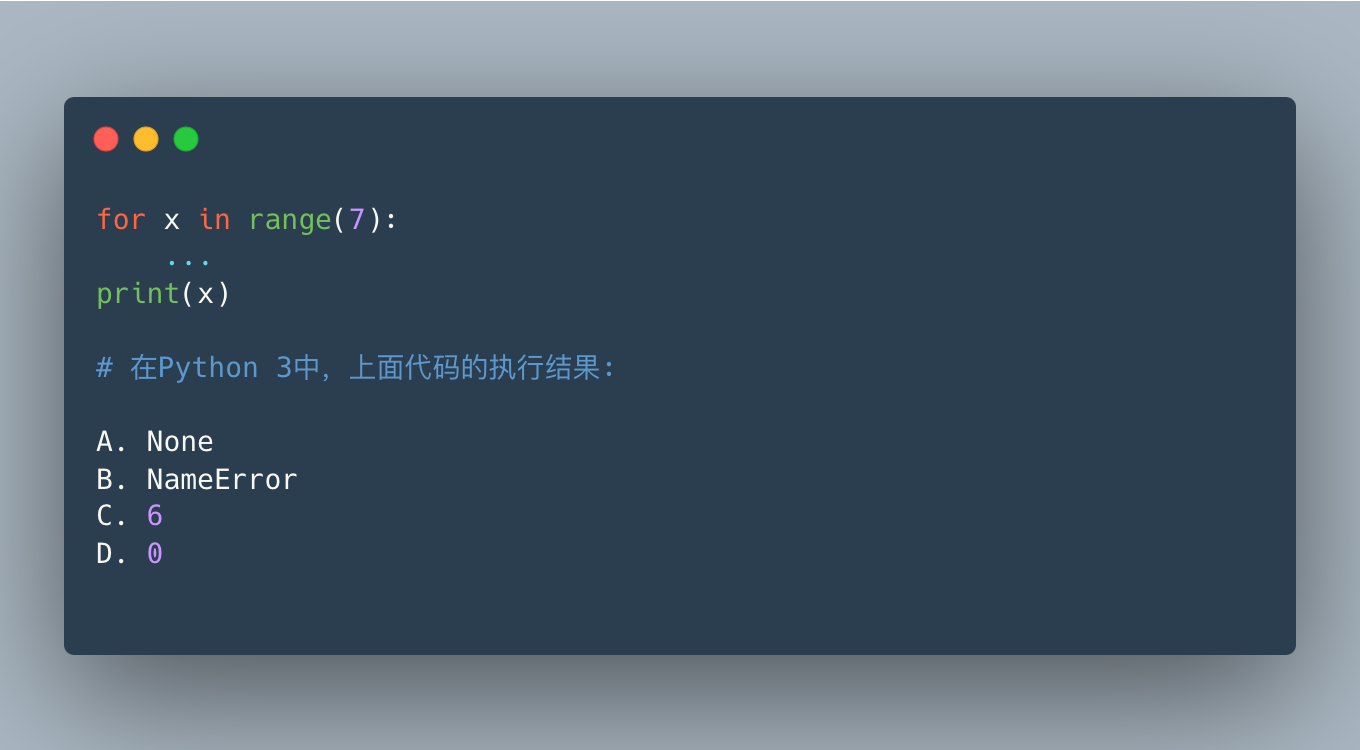For the sub-question, the answer is C. The for loop will affect the value of the variable outside the scope, but one thing to note, starting with Python 3, the list comprehension will not affect the value of the variable outside the scope, for example:

 ` In : x = 1 In : print ([ x for x in range ( 5 )]) [ 0 , 1 , 2 , 3 , 4 ] In : x Out : 1 # not affected by list comprehension `

### topic 19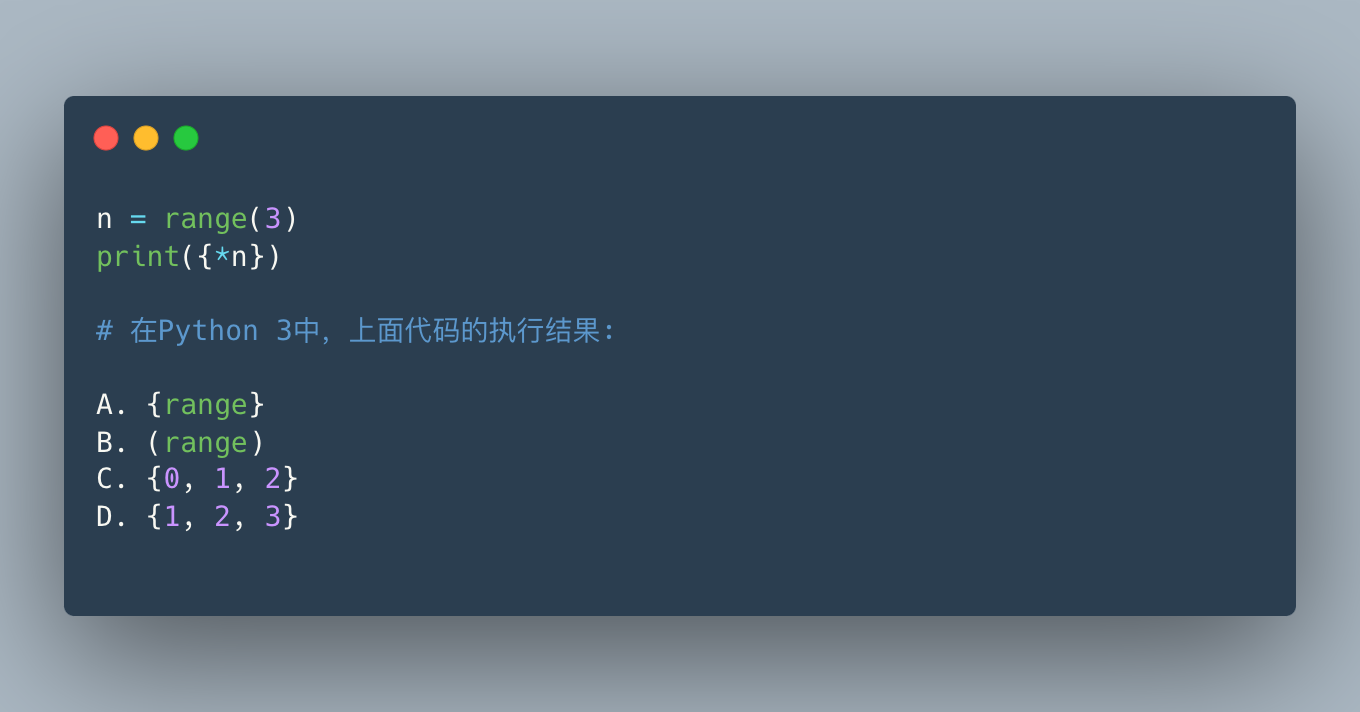Absolutely give points, the answer is C. However, I didn’t write this question well. The original title will automatically remove A/B2 answers for developers who are familiar with Python. The options should be:

 ` A. { range ( 0 , 3 ) } B. ( range ( 0 , 3 ) ) C . { 0 , 1 , 2 } D. { 1 , 2 , 3 } _ `

This makes it even more confusing. In fact, it is to unpack an iterable object of type range in the collection.

### topic 20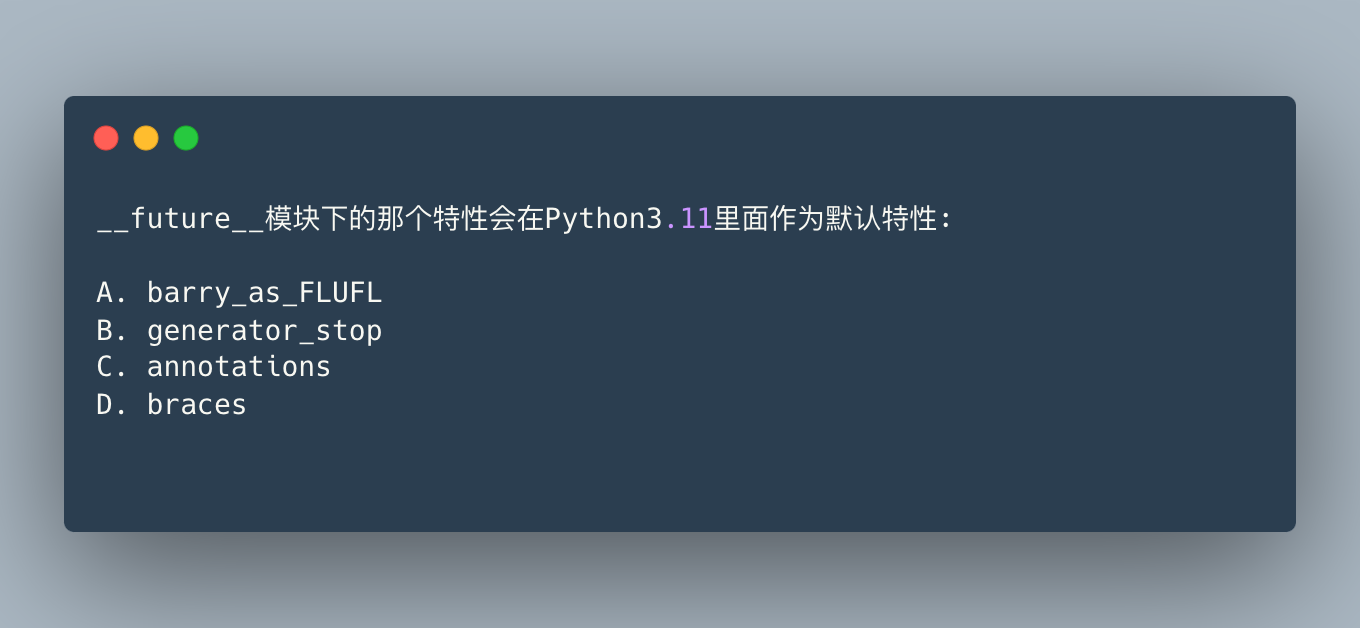This is actually a language design problem, the answer is C.

First of all, `yield from` is actually:

 ` for i in range ( x ): yield i `

the meaning of. It mainly tests your familiarity with generators and `yield from` , which is newly added in Python 3.3. If a function has `yield` or `yield from` , then this is a generator:

 ` In : def a (): ... : yield 1 ... : In : a () Out : < generator object a at 0x1076aa040 > In : def b (): ... : yield from [ 1 , 2 ] ... : In : b () Out : < generator object b at 0x1051af820 > `

At the time of design, the generator can be used return , which is actually the purpose of stopping the generator, as the official said:

“… return expr in a generator causes StopIteration(expr) to be raised upon exit from the generator.”

So `return ['wtf']` is equal to `raise StopIteration(['wtf'])` , and when `list()` is executed, it will catch the error and end directly.

But in our example, the directly matched return condition causes it to directly return an empty list (because StopIteration is raised as soon as it comes up).

### topic 21

Finally, look at the 10 questions in National Volume C. This is also the hardest one in my opinion.To test your knowledge of the `__future__` module, the answer is C. Everyone should understand the meaning of each option, and I will not mention them one by one here.

### topic 22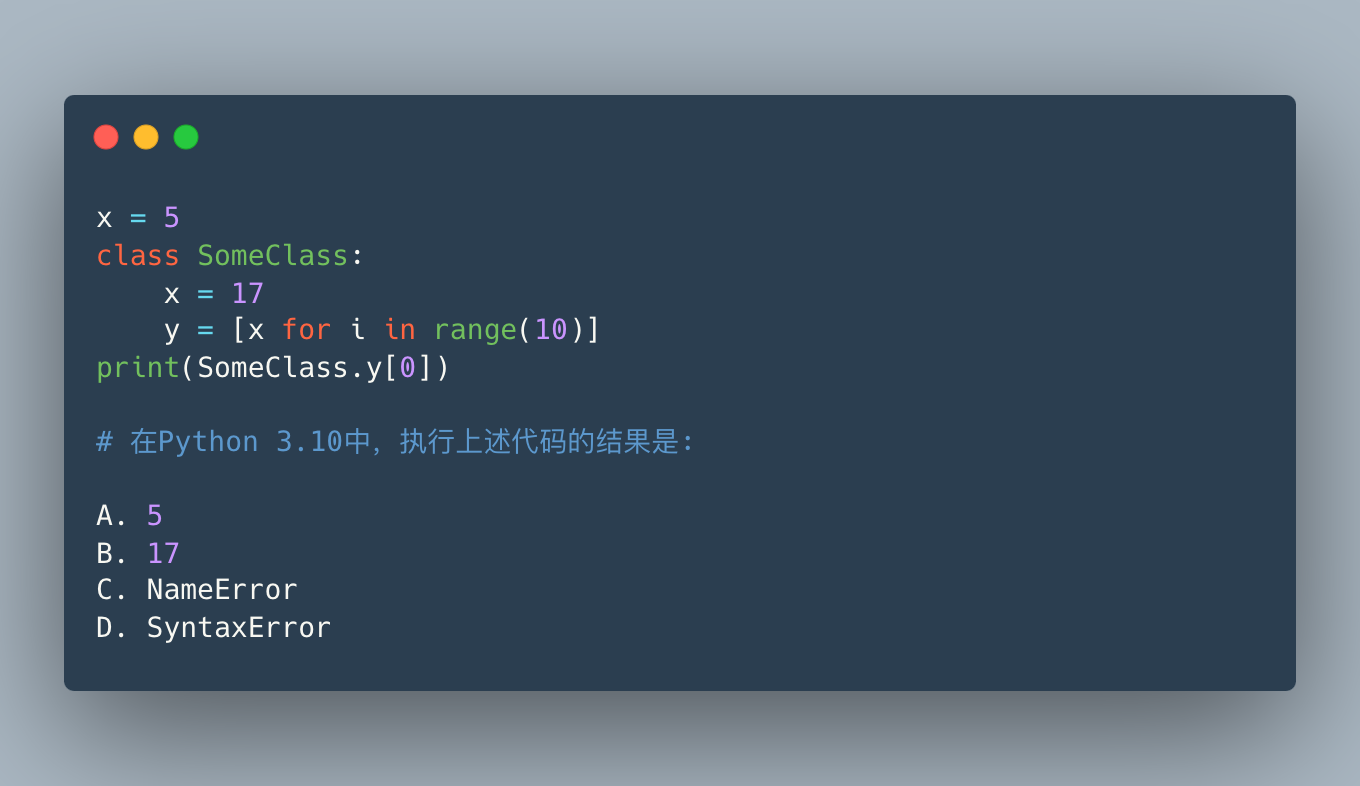The answer is A. First, a scope nested within a class definition ignores variables bound at the class level.

Also, as mentioned in Topic 18, Python 3’s list comprehension/generator has its own scope, and doesn’t affect the outside world.

### topic 23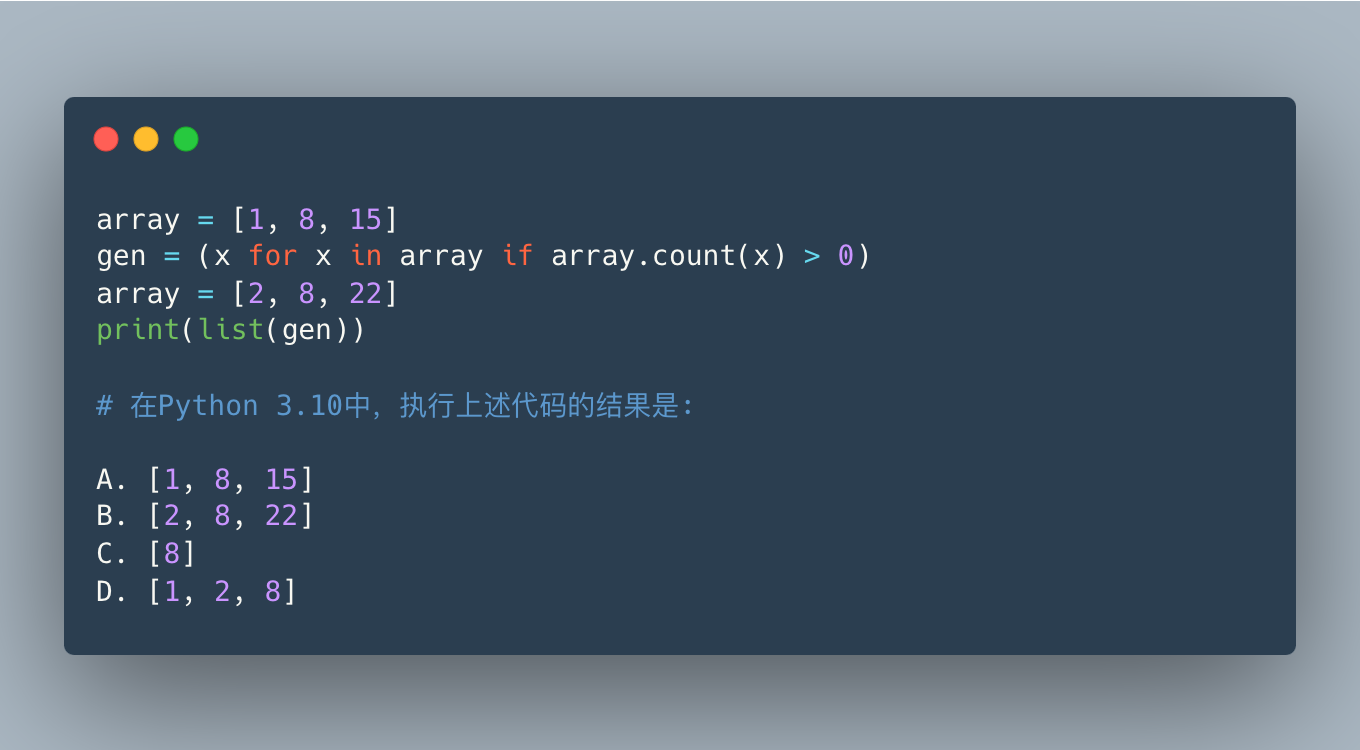The answer is C. In a generator expression, `in` is the declaration-time evaluation, and the conditional evaluation is performed at runtime. So in this question `gen = (x for x in array if array.count(x) > 0)` is `gen = (x for x in [1, 8, 15] if [2, 8, 22].count(x) > 0)`

This place needs everyone to understand carefully.

### topic 24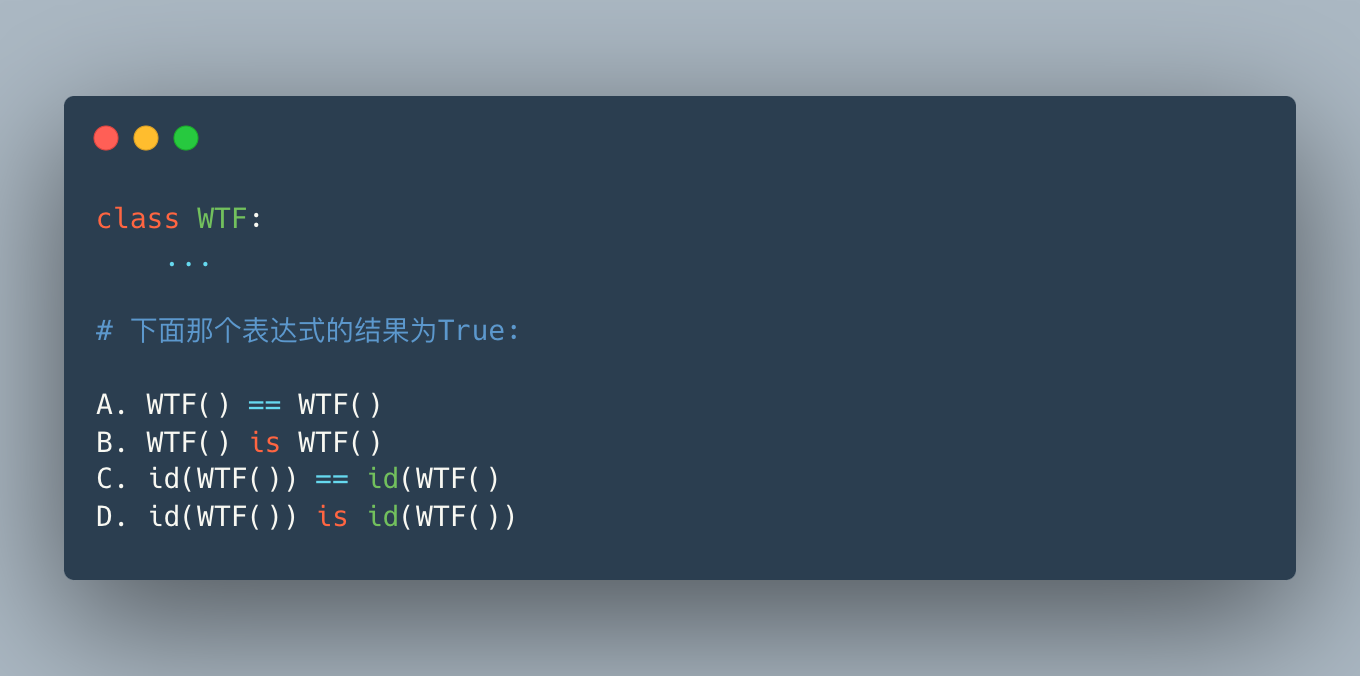Topic inspiration: https://github.com/satwikkansal/wtfpython#-deep-down-were-all-the-same

Let’s first think about the relationship between `==` and `is` : `==` means the value is equal, and `is` means the content address pointed to is the same, so the two options for comparison may be `==` but may not be `is` , because `is` requires higher requirements, the same, if `is` , then definitely `==` .

Let’s check one by one. `WTF() == WTF()` means that 2 instances are definitely not the same; since `WTF() is WTF()` are not equal, `is` even more impossible.

If you understand the relationship between `==` and `is` , you can now know that the answer is C. But why is `id(WTF()) == id(WTF())` and `id(WTF()) is id(WTF())` wrong?

In an expression, if the id function is executed 2 times, the latter one will be allocated to the same memory location, so equal `==` . But `id(WTF()) is id(WTF())` has little to do with this topic. It is an option I play. Here is a more direct example:

 ` In : a = id ( 0 ) In : b = id ( 0 ) In : a Out : 4373539088 In : b Out : 4373539088 In : a is b Out : False In : id ( 0 ) is id ( 0 ) Out : False `

This is a pooling problem. If the number is not between – 5 and 256, Python will not cache the number object, and the result of the id function execution is much larger than this value, so it is False.

Let’s try it on the latest Python 3.10. The default output does not meet expectations. I’ll change my mind:

 ` In : 256 is 256 # Error output <> : 1 : SyntaxWarning : "is" with a literal . Did you mean "==" ? < ipython - input - 1 - 975396 cd1f1b > : 1 : SyntaxWarning : "is" with a literal . Did you mean "==" ? 256 is 256 Out : True In : 257 is 257 # error output <> : 1 : SyntaxWarning : "is" with a literal . Did you mean "==" ? < ipython - input - 2 - dd70d8dec340 > : 1 : SyntaxWarning : "is" with a literal . Did you mean "==" ? 257 is 257 Out : True In : def add ( n ): # Let's change our mind ... : return n + 256 ... : In : add ( 0 ) is add ( 0 ) # 256 is 256 Out : True In : add ( 1 ) is add ( 1 ) # 257 is 257 Out : False # more than 256 so it is False `

### topic 25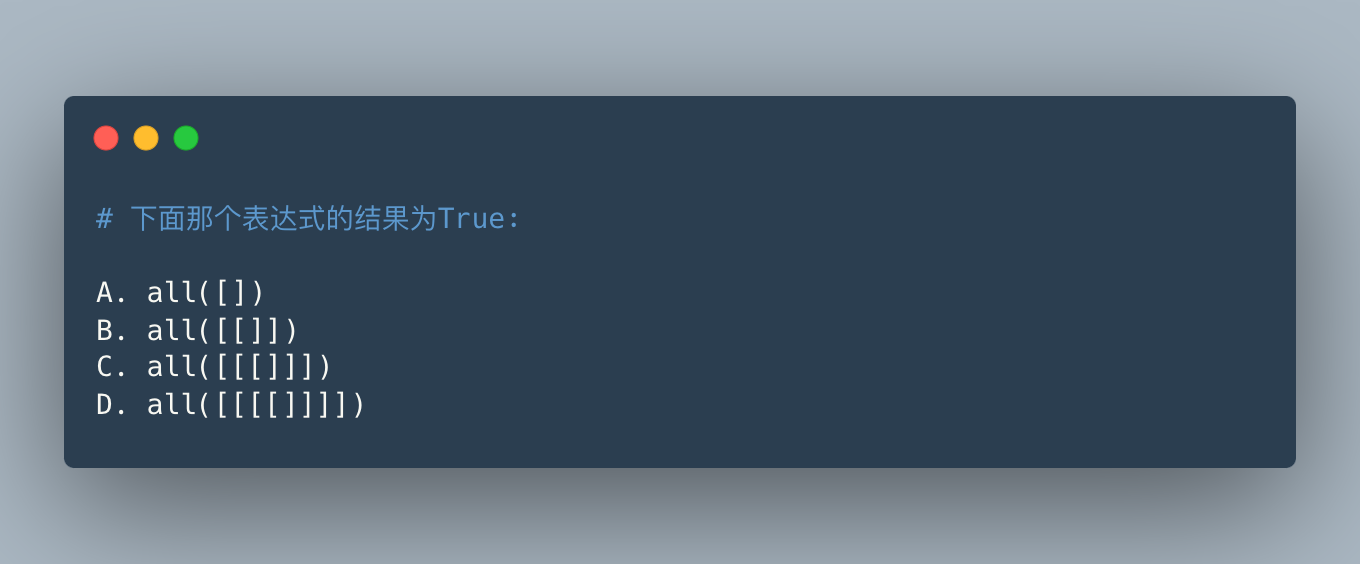In fact, careful analysis can find the answer, the answer is B.

`all` means to loop the parameters, and each element meets the requirements to be True, and as long as one does not meet the requirements, it is False. So `all([])` is an empty list and the result is empty, `all([[]])` means that the list has only one element `[]` , and the empty list is False, so the result is False.

The last 2 options are a little confusing, the list has only one element, `[[]]` and `[[[]]]` , they are both non-empty, so the boolean value is True:

 ` In : bool ([[]]) Out : True In : bool ([[[]]]) Out : True `

### Topic 26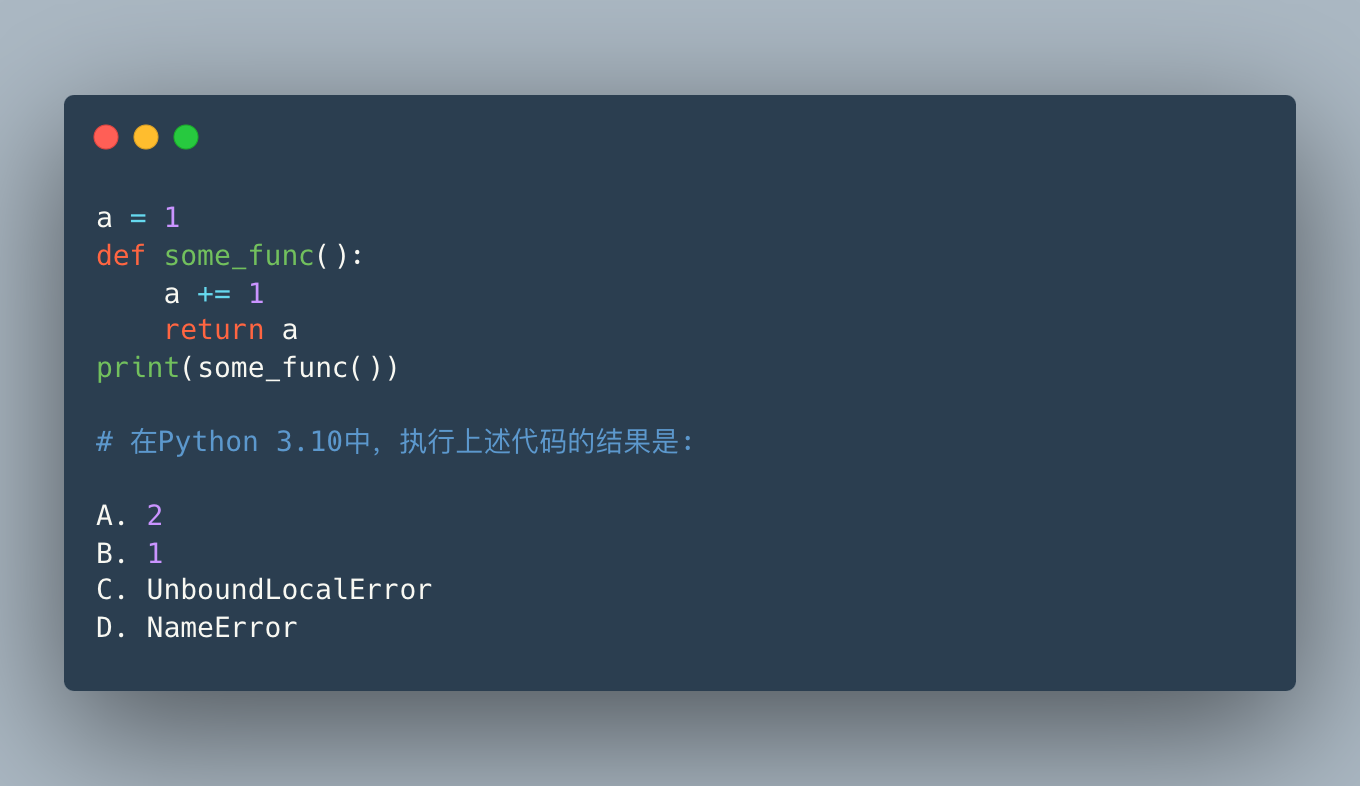This is relatively simple, senior Python developers should have written such code, and the answer is C.

In Python, if you assign a value to a variable, then this variable will program the local variable of the current scope, so in the function `some_func` `a += 1` , `a` becomes a local variable in the function, but in the function scope, there is no previous Define a or assign a value to a, only `a += 1` , so UnboundLocalError is thrown.

If you want the program not to report errors, the solution is to add the global keyword to the function:

 ` In : a = 1 In : def some_func (): ... : global a ... : a += 1 ... : return a ... : In : some_func () Out : 2 `

Note, however, that global should be used with caution, as its name implies, use of it will affect the result of the global variable.

### topic 27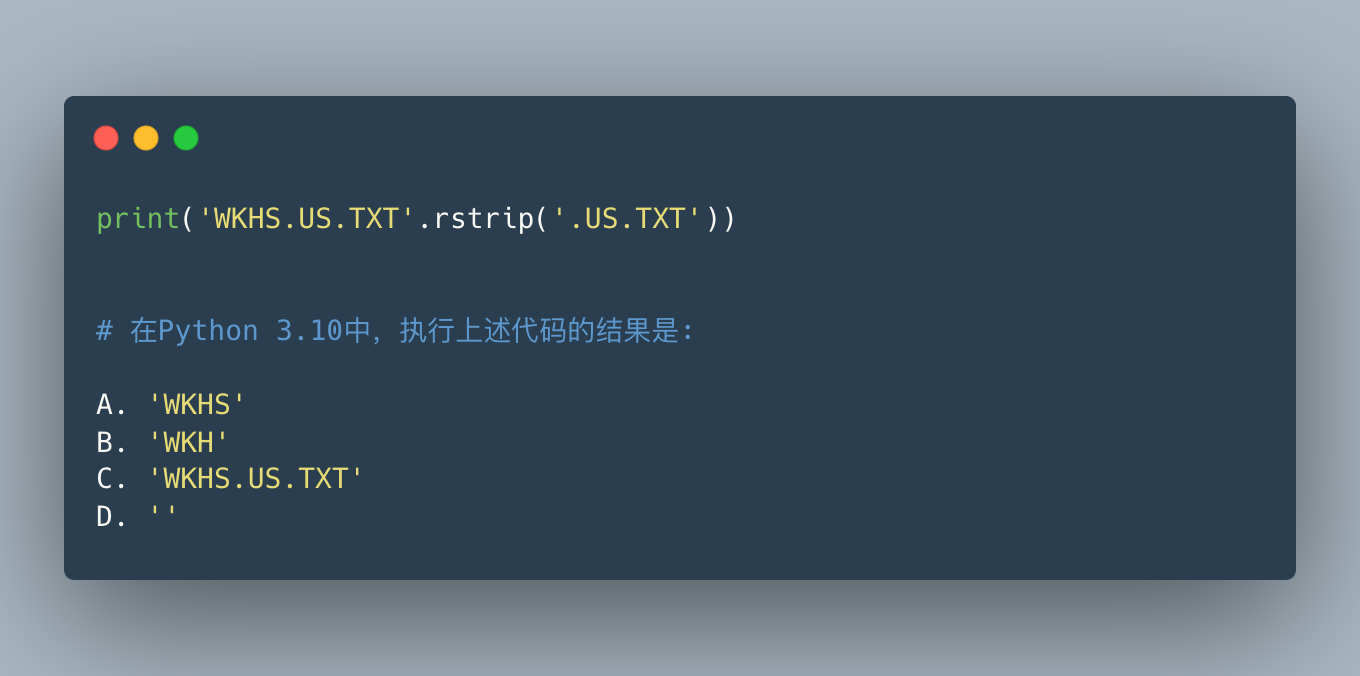The answer is B. This question examines the meaning of methods such as `strip` and `rstrip` for strings. By default they are used to strip whitespace from string lines:

 ` In : ' s ' . strip () Out : 's' In : ' s ' . rstrip () Out : 's' In : ' s ' . lstrip () Out : 's ' `

But you can also pass in other strings to implement what the `replace` function does to replace the corresponding match with empty:

 ` In : ' s ' . rstrip ( 's' ) Out : ' s ' In : ' s ' . rstrip ( 's ' ) Out : '' In : 'abc' .rstrip ( ' bc' ) Out : 'a' `

Therefore, the `.US.TXT` contained in the original string will be replaced, that is, the characters `. USTX` will be replaced with empty.

Note that if you just want to remove the suffix, you can use the `removesuffix` / `removeprefix` newly added in Python 3.9:

 ` In : 'WKHSS.US.TXT' . removesuffix ( '.US.TXT' ) Out : 'WKHSS' `

### topic 28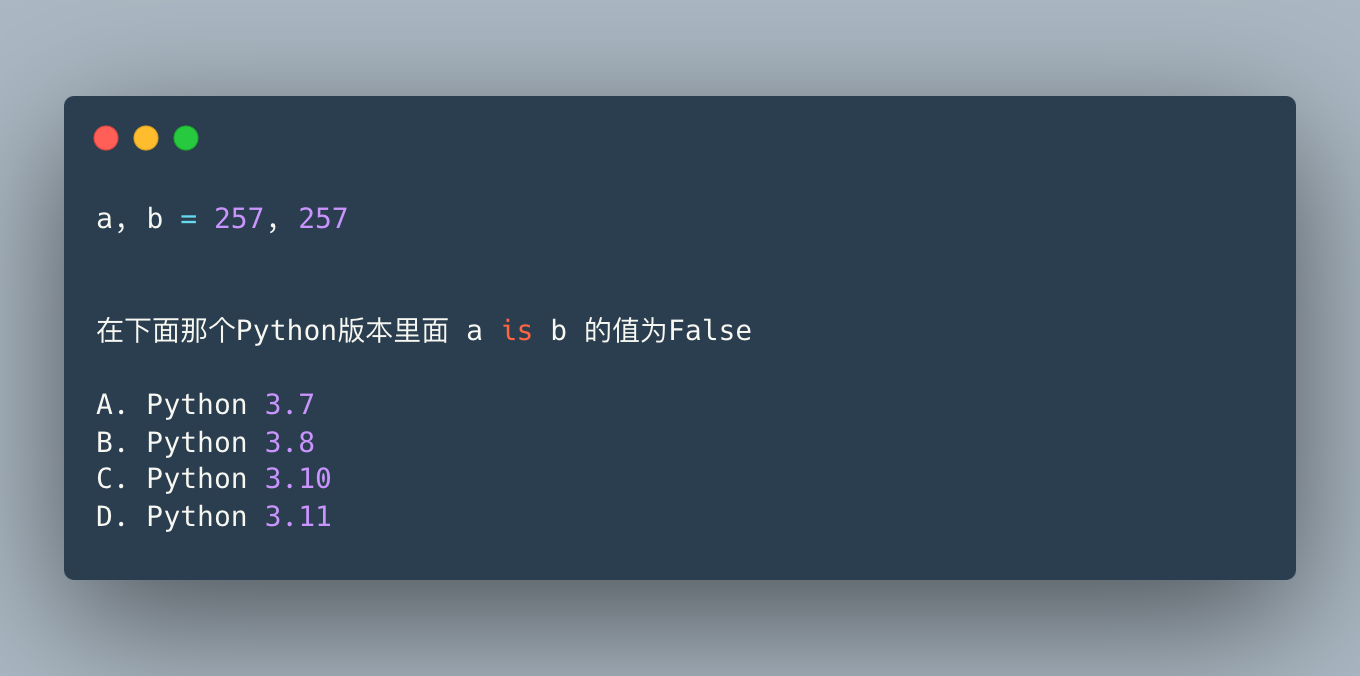The answer is A. This is actually a bug in Python 3.7, which has been fixed in https://bugs.python.org/issue34100 .

### topic 29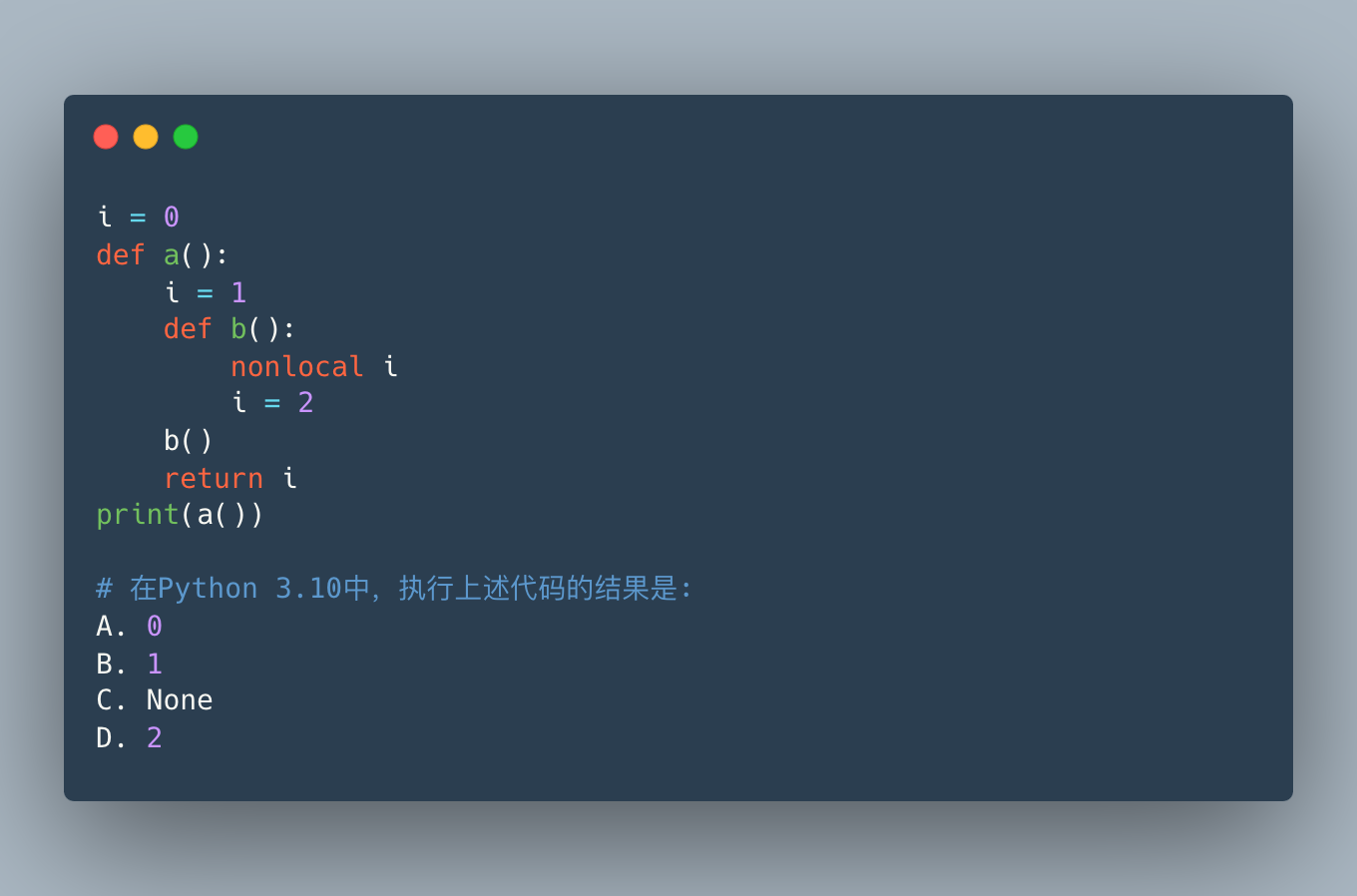This question tests the understanding of `nonlocal` , and the answer is D. We have also covered it in the previous topic. Let’s look at an example first:

 ` In : i = 0 ... : ... : def a (): ... : i = 1 ... : print ( 'local:' , i ) ... : ... : a () local : 1 In : i Out : 0 `

This is expected, the assignment to i within the function scope is local and does not affect the global variable outside. The global scheme mentioned earlier in Topic 26 can affect external global variables, but what about nested scopes:

“` python
I…